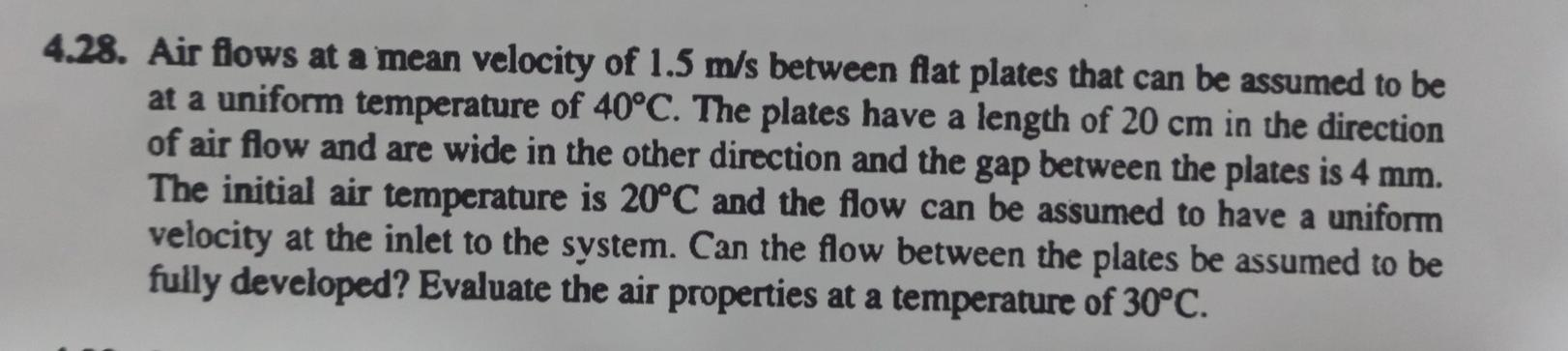# Question Solved1 Answer4.28. Air flows at a mean velocity of 1.5 m/s between flat plates that can be assumed to be at a uniform temperature of 40°C. The plates have a length of 20 cm in the direction of air flow and are wide in the other direction and the gap between the plates is 4 mm. The initial air temperature is 20°C and the flow can be assumed to have a uniform velocity at the inlet to the system. Can the flow between the plates be assumed to be fully developed? Evaluate the air properties at a temperature of 30°C.OH8RWD The Asker · Mechanical EngineeringTranscribed Image Text: 4.28. Air flows at a mean velocity of 1.5 m/s between flat plates that can be assumed to be at a uniform temperature of 40°C. The plates have a length of 20 cm in the direction of air flow and are wide in the other direction and the gap between the plates is 4 mm. The initial air temperature is 20°C and the flow can be assumed to have a uniform velocity at the inlet to the system. Can the flow between the plates be assumed to be fully developed? Evaluate the air properties at a temperature of 30°C.
More
Transcribed Image Text: 4.28. Air flows at a mean velocity of 1.5 m/s between flat plates that can be assumed to be at a uniform temperature of 40°C. The plates have a length of 20 cm in the direction of air flow and are wide in the other direction and the gap between the plates is 4 mm. The initial air temperature is 20°C and the flow can be assumed to have a uniform velocity at the inlet to the system. Can the flow between the plates be assumed to be fully developed? Evaluate the air properties at a temperature of 30°C.